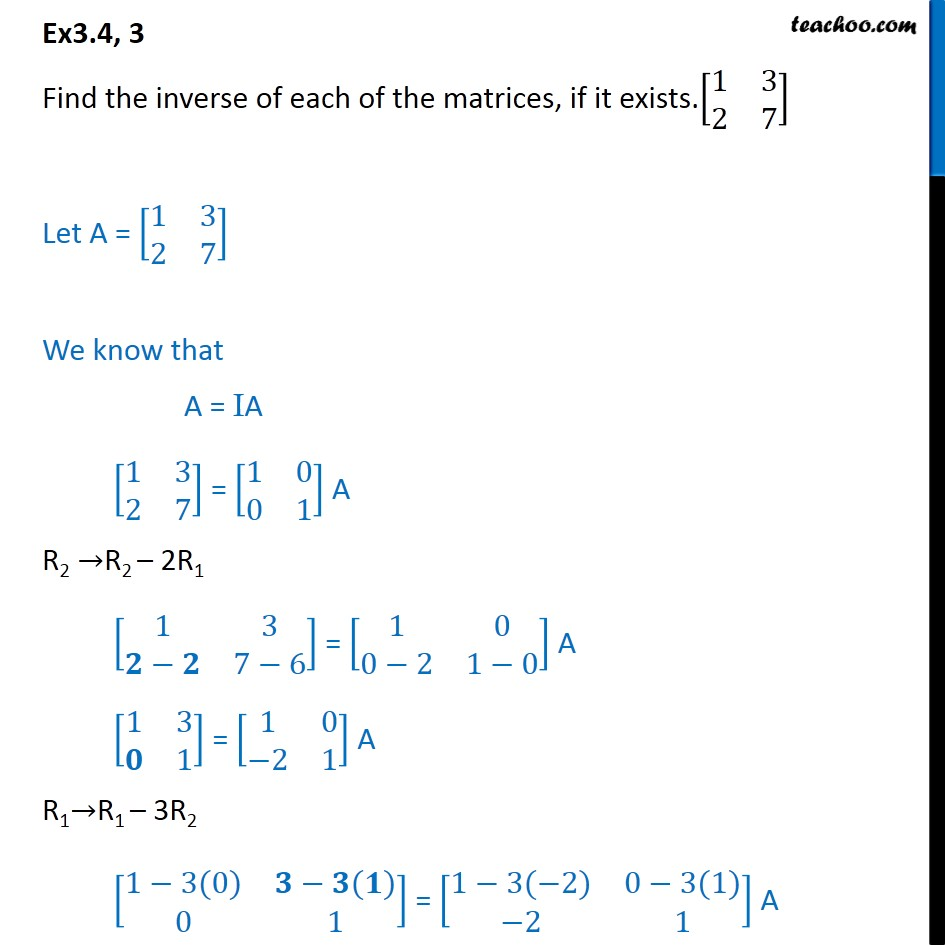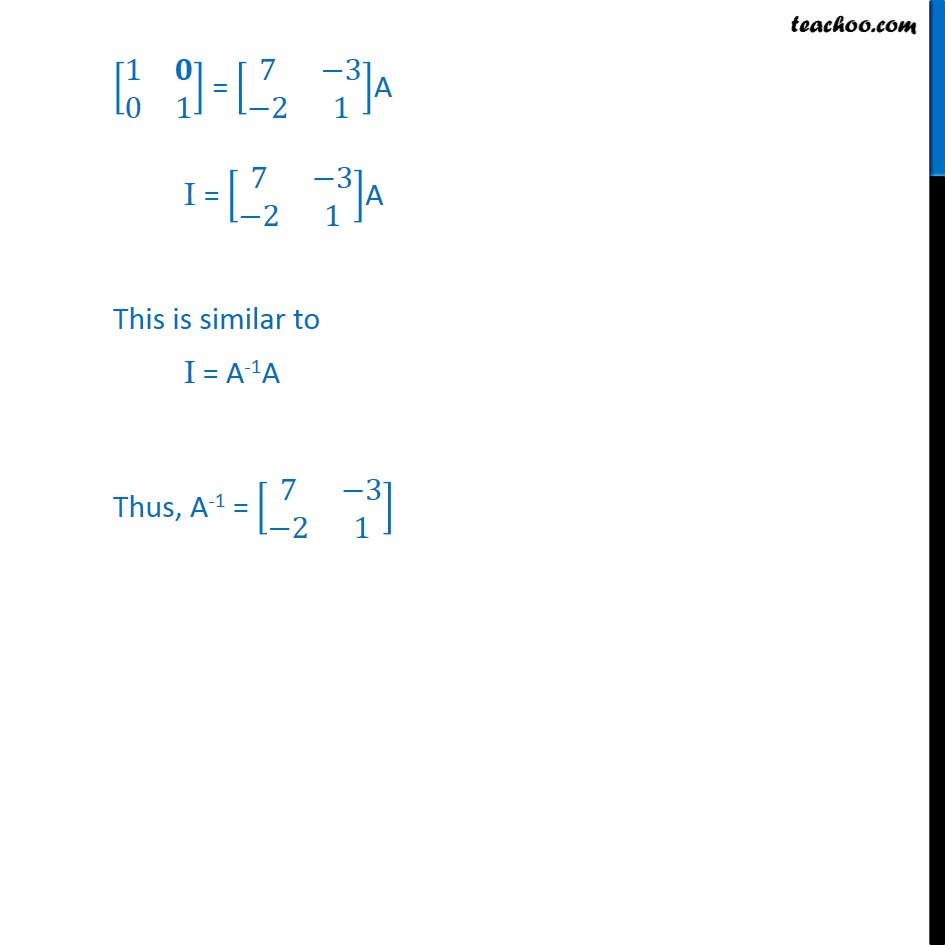1. Chapter 3 Class 12 Matrices
2. Concept wise
3. Inverse of matrix using elementary transformation

Transcript

Ex3.4, 3 Find the inverse of each of the matrices, if it exists.[■8(1&3@2&7)] Let A = [■8(1&3@2&7)] We know that A = IA [■8(1&3@2&7)] = [■8(1&0@0&1)] A R2 →R2 – 2R1 [■8(1&3@𝟐−𝟐&7−6)] = [■8(1&0@0−2&1−0)] A [■8(1&3@𝟎&1)] = [■8(1&0@−2&1)] A R1→R1 – 3R2 [■8(1−3(0)&𝟑−𝟑(𝟏)@0&1)] = [■8(1−3(−2)&0−3(1)@−2&1)] A [■8(1&𝟎@0&1)] = [■8(7&−3@−2&1)]A I = [■8(7&−3@−2&1)]A This is similar to I = A-1A Thus, A-1 = [■8(7&−3@−2&1)]

Inverse of matrix using elementary transformation